# Jointly distributed Random variables Multivariate distributions Discrete Random

• Slides: 49Jointly distributed Random variables Multivariate distributionsDiscrete Random VariablesThe joint probability function; p(x, y) = P[X = x, Y = y]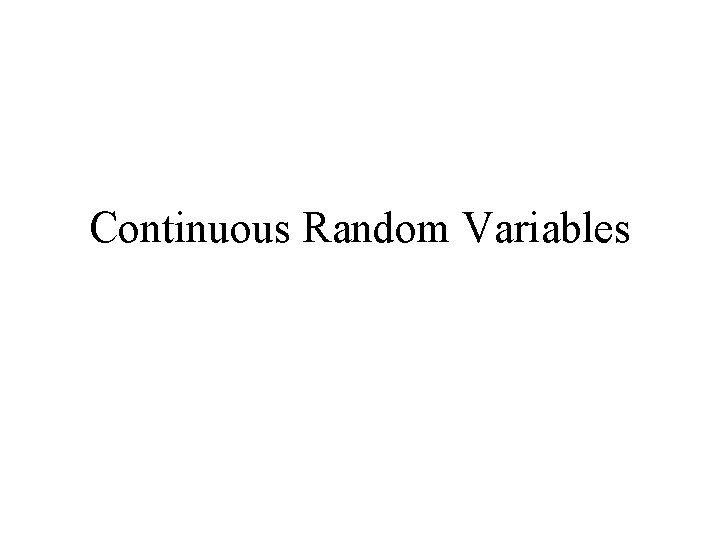Continuous Random VariablesDefinition: Two random variable are said to have joint probability density function f(x, y) ifIf then defines a surface over the x – y planeMultiple Integration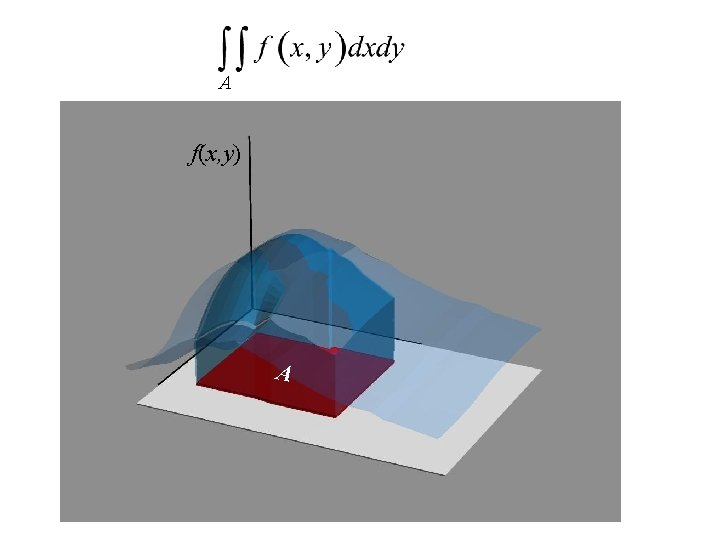A f(x, y) A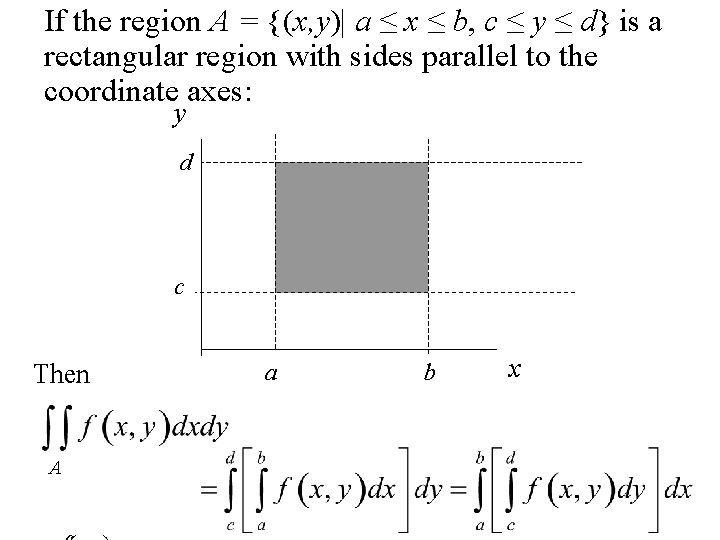If the region A = {(x, y)| a ≤ x ≤ b, c ≤ y ≤ d} is a rectangular region with sides parallel to the coordinate axes: y d c Then A a b xTo evaluate A First evaluate the inner integral Then evaluate the outer integral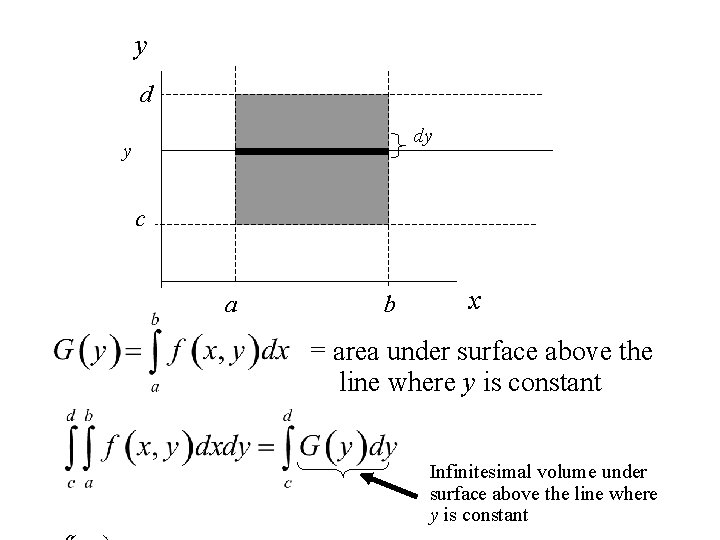y d dy y c a b x = area under surface above the line where y is constant Infinitesimal volume under surface above the line where y is constant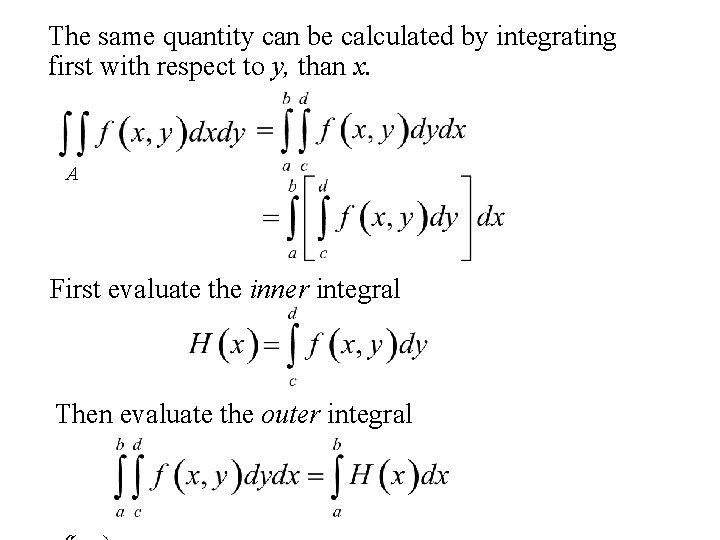The same quantity can be calculated by integrating first with respect to y, than x. A First evaluate the inner integral Then evaluate the outer integral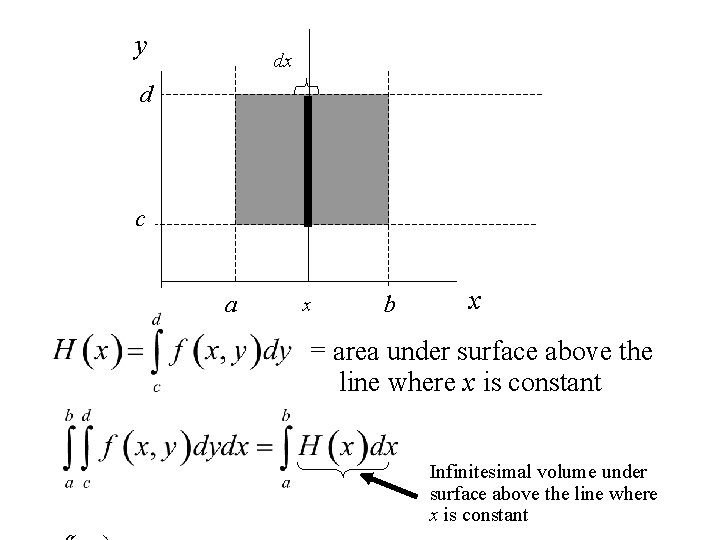y dx d c a x b x = area under surface above the line where x is constant Infinitesimal volume under surface above the line where x is constant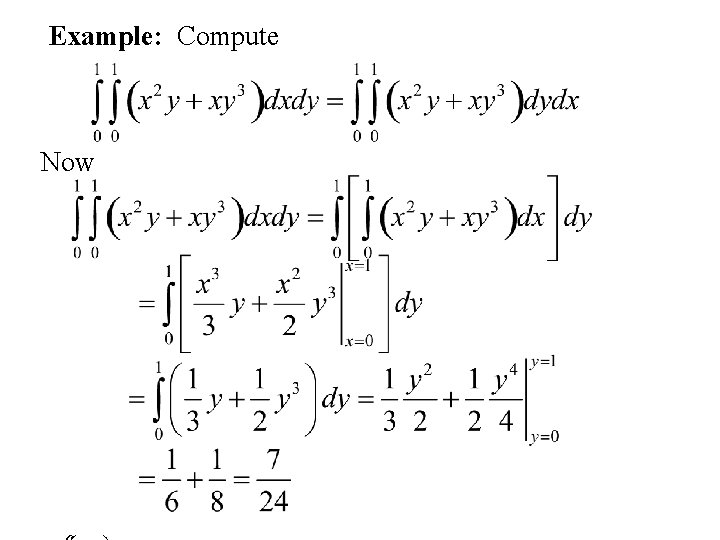Example: Compute Now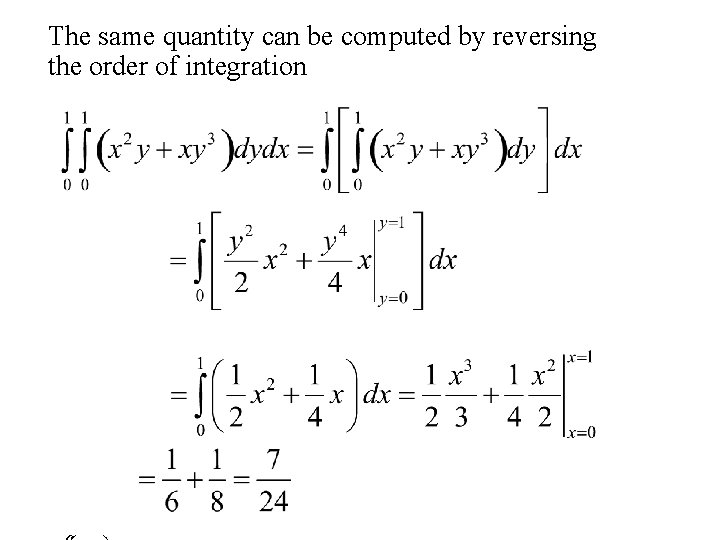The same quantity can be computed by reversing the order of integration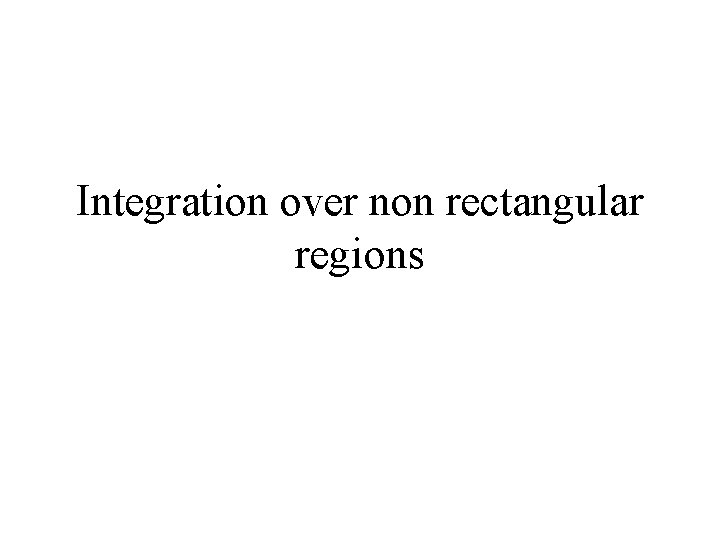Integration over non rectangular regions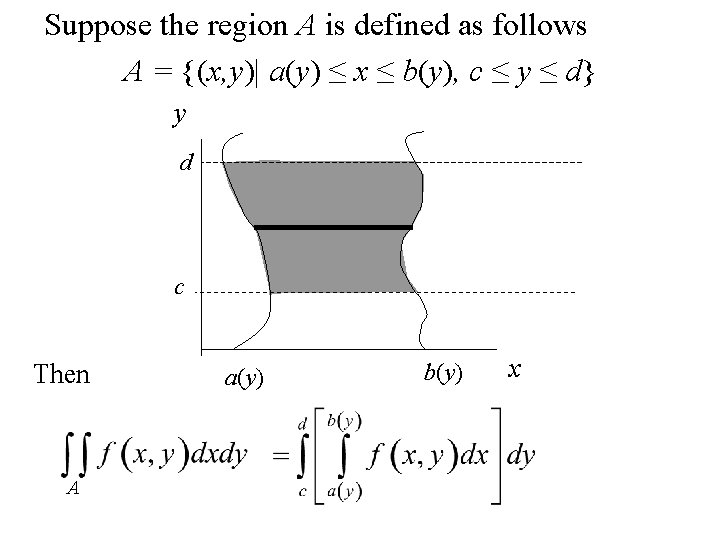Suppose the region A is defined as follows A = {(x, y)| a(y) ≤ x ≤ b(y), c ≤ y ≤ d} y d c Then A a(y) b(y) x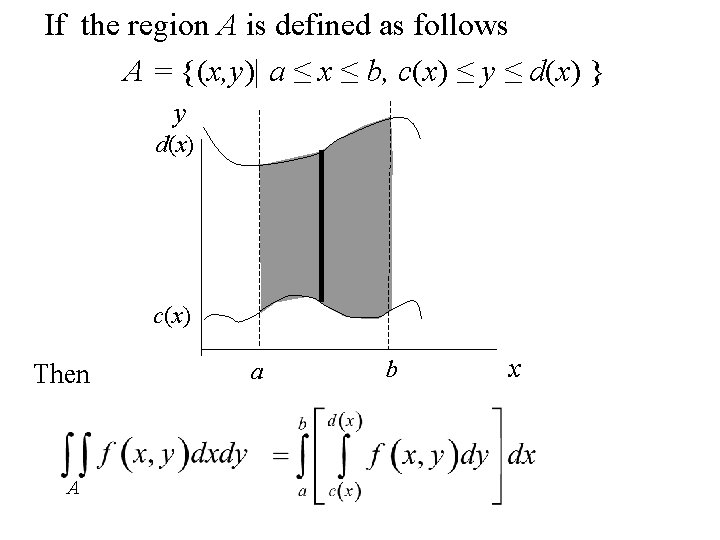If the region A is defined as follows A = {(x, y)| a ≤ x ≤ b, c(x) ≤ y ≤ d(x) } y d(x) c(x) Then A a b x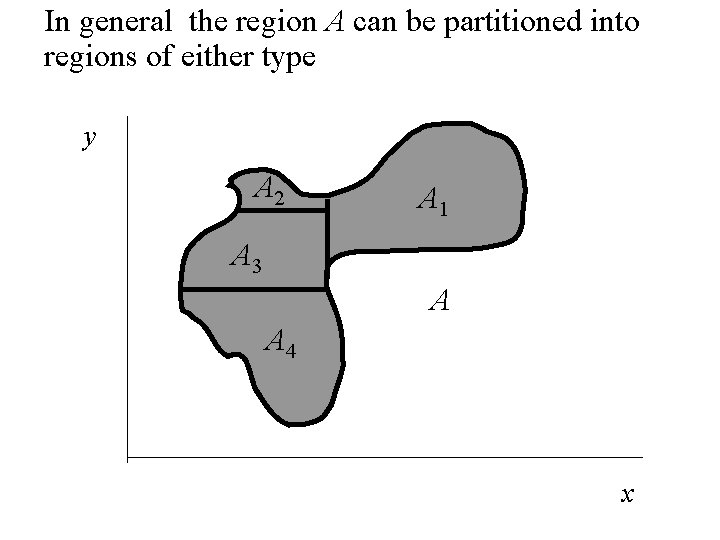In general the region A can be partitioned into regions of either type y A 2 A 1 A 3 A A 4 xExample: Compute the volume under f(x, y) = x 2 y + xy 3 over the region A = {(x, y)| x + y ≤ 1, 0 ≤ x, 0 ≤ y} y (0, 1) x+y=1 (1, 0) xIntegrating first with respect to x than y y (0, 1) (0, y) x+y=1 (1 - y, y) (1, 0) x AandNow integrating first with respect to y than x y (0, 1) x+y=1 (x, 1 – x ) (1, 0) (x, 0) A x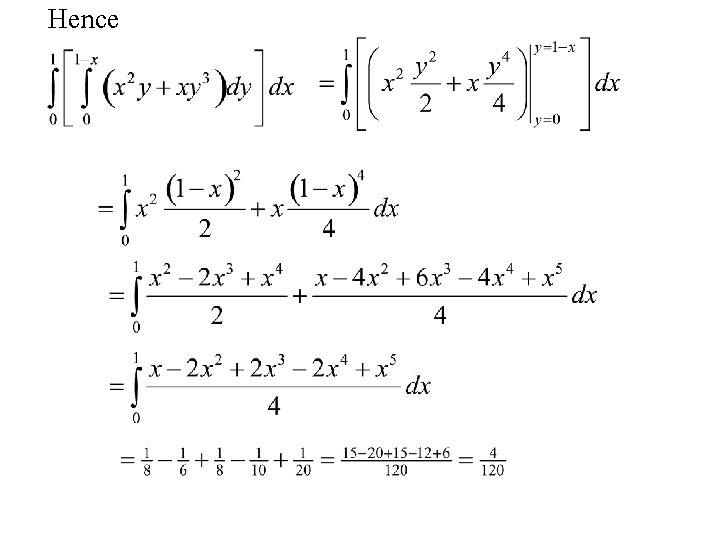HenceContinuous Random Variables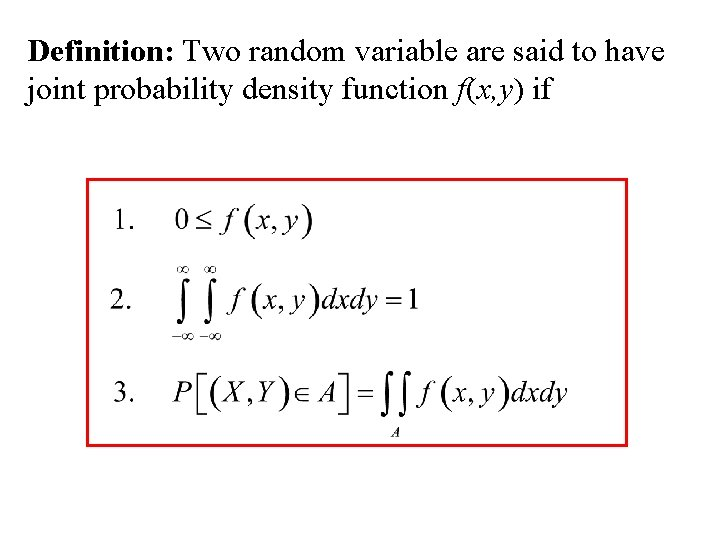Definition: Two random variable are said to have joint probability density function f(x, y) if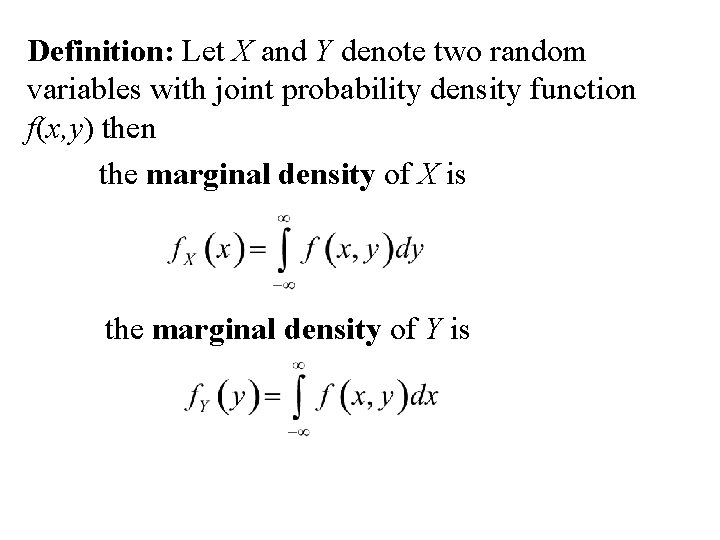Definition: Let X and Y denote two random variables with joint probability density function f(x, y) then the marginal density of X is the marginal density of Y is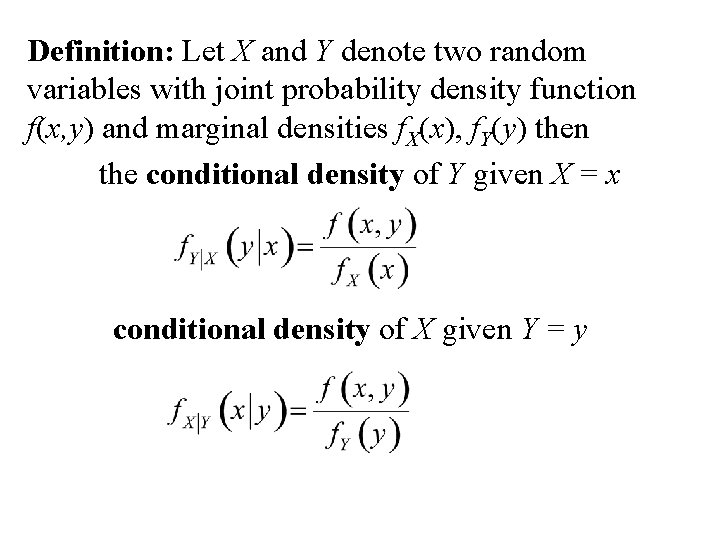Definition: Let X and Y denote two random variables with joint probability density function f(x, y) and marginal densities f. X(x), f. Y(y) then the conditional density of Y given X = x conditional density of X given Y = yThe bivariate Normal distribution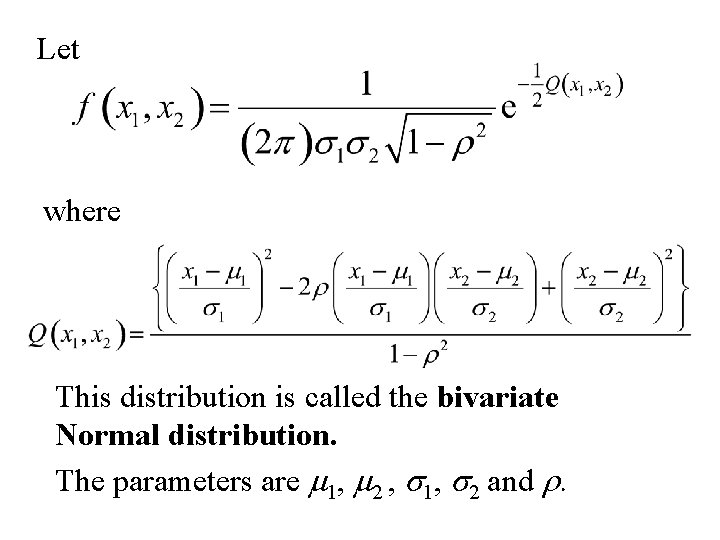Let where This distribution is called the bivariate Normal distribution. The parameters are m 1, m 2 , s 1, s 2 and r.Surface Plots of the bivariate Normal distributionNote: is constant when is constant. This is true when x 1, x 2 lie on an ellipse centered at m 1, m 2.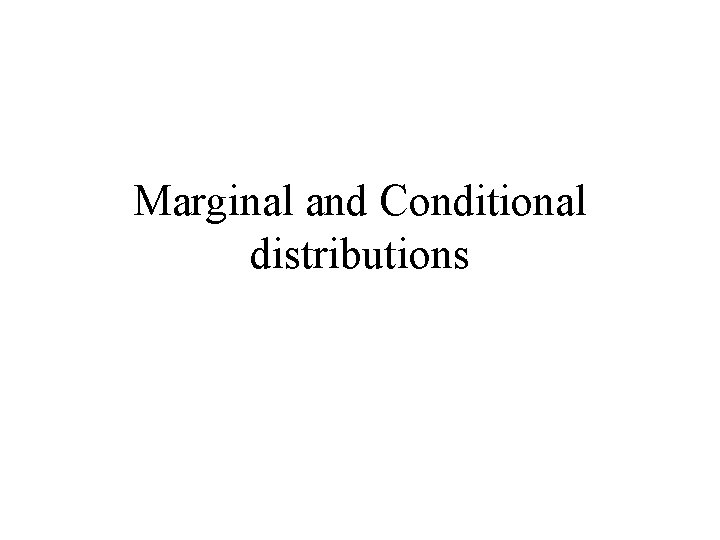Marginal and Conditional distributions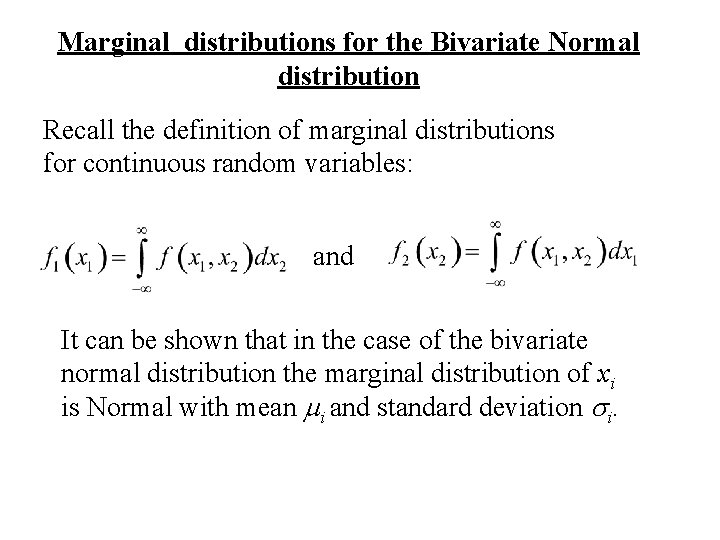Marginal distributions for the Bivariate Normal distribution Recall the definition of marginal distributions for continuous random variables: and It can be shown that in the case of the bivariate normal distribution the marginal distribution of xi is Normal with mean mi and standard deviation si.Proof: The marginal distributions of x 2 is where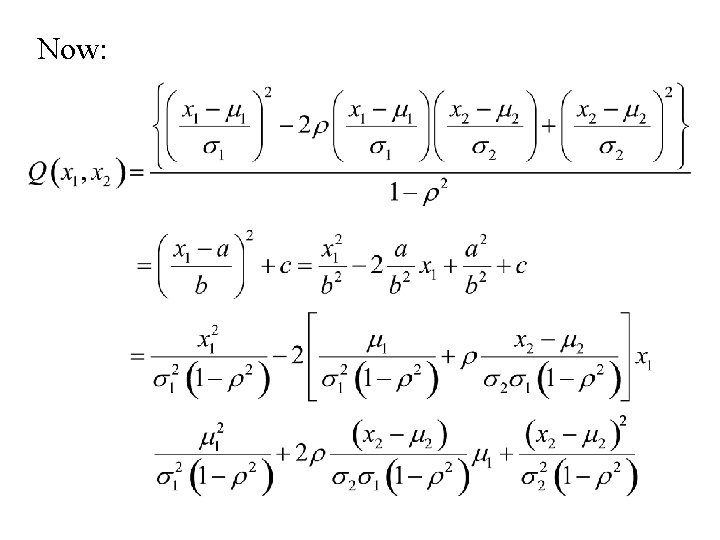Now: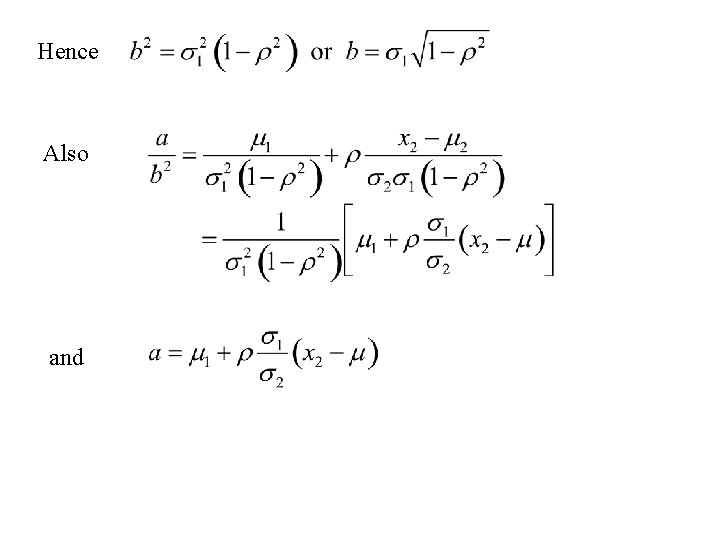Hence Also andFinally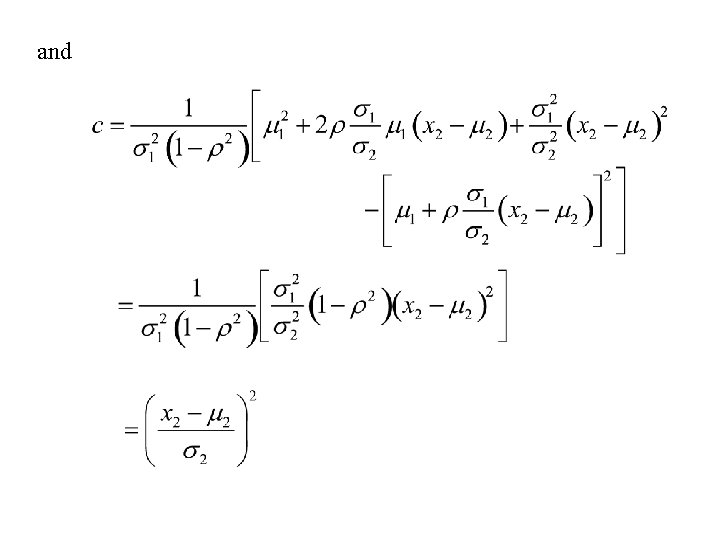andSummarizing where and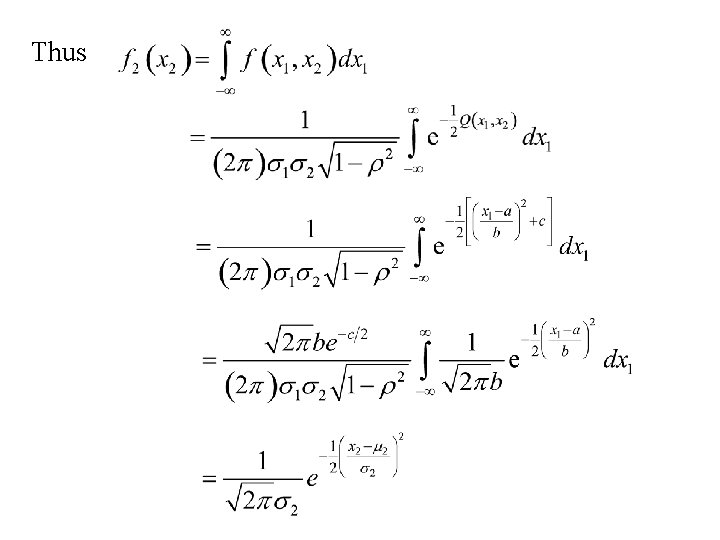Thus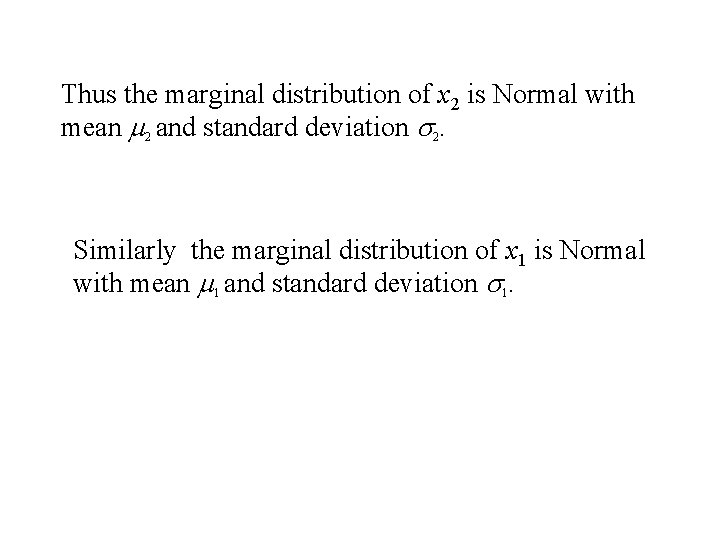Thus the marginal distribution of x 2 is Normal with mean m 2 and standard deviation s 2. Similarly the marginal distribution of x 1 is Normal with mean m 1 and standard deviation s 1.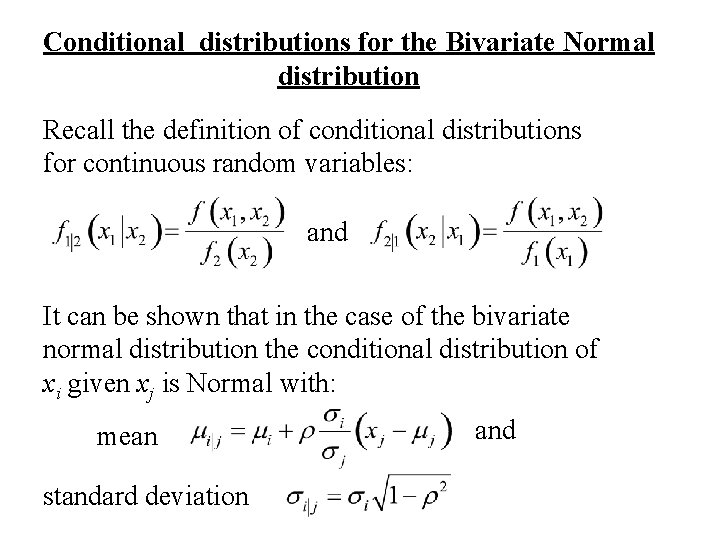Conditional distributions for the Bivariate Normal distribution Recall the definition of conditional distributions for continuous random variables: and It can be shown that in the case of the bivariate normal distribution the conditional distribution of xi given xj is Normal with: mean standard deviation and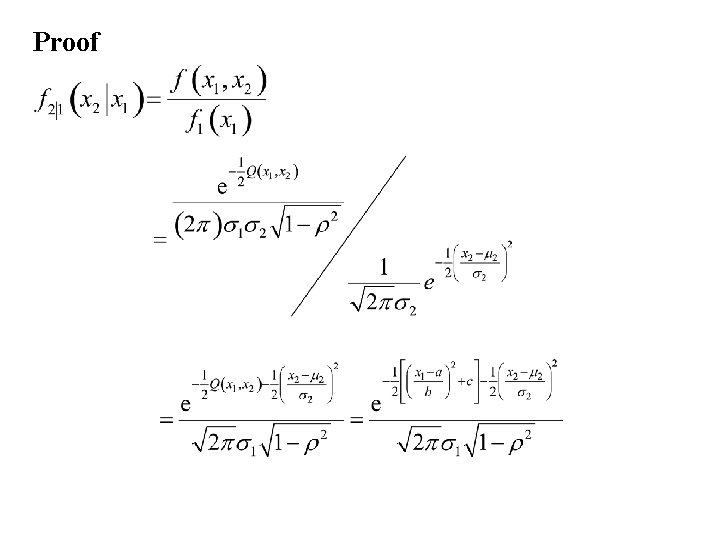Proof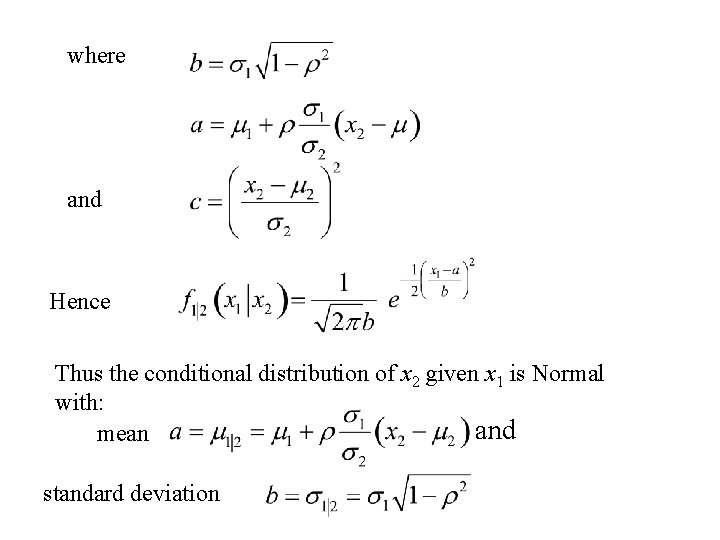where and Hence Thus the conditional distribution of x 2 given x 1 is Normal with: and mean standard deviation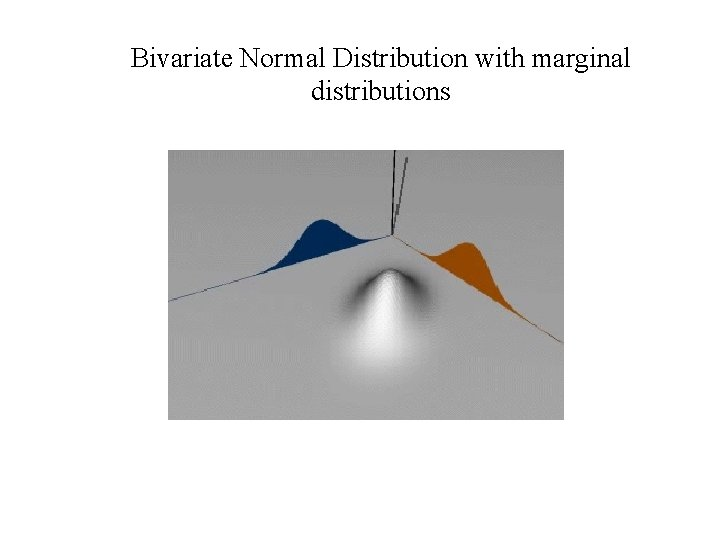Bivariate Normal Distribution with marginal distributions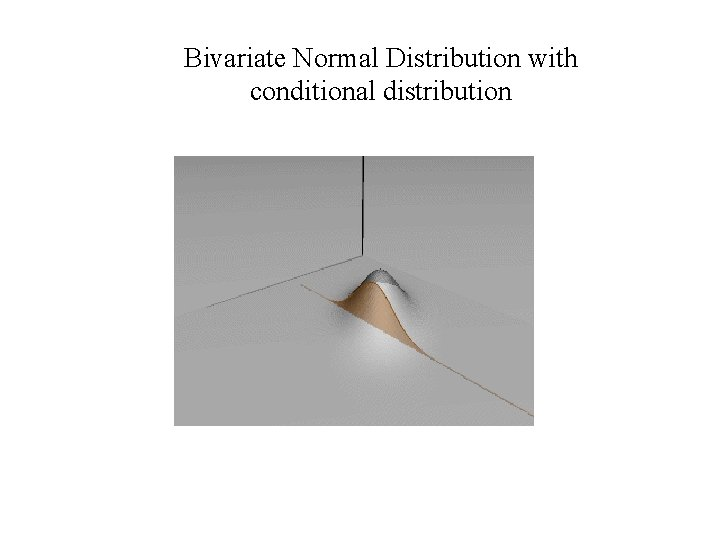Bivariate Normal Distribution with conditional distribution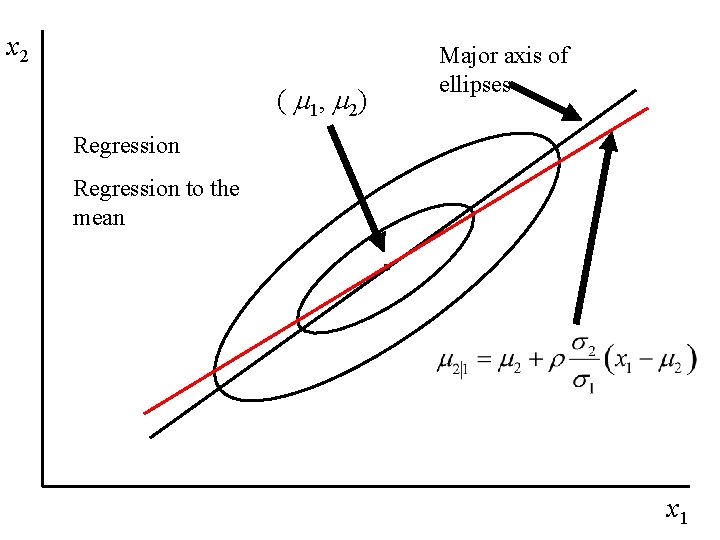x 2 ( m 1, m 2) Major axis of ellipses Regression to the mean x 1